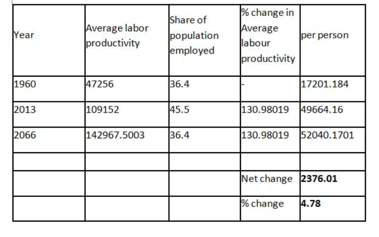# The ?graying of America? will substantially increase the fraction of the population that is...

## Question:

The ?graying of America? will substantially increase the fraction of the population that is retired in the decades to come. To illustrate the implications for U.S. living standards, suppose that over the 53 years following 2013 the share of the population that is working returns to its 1960 level, while average labor productivity increases at the same rate as it did during 1960?2013. Under this scenario, what would be the net change in real GDP per person between 2013 and 2066?

Year Average labor productivity Share of population employed
1960 47,256 36.4%
2013 109.152 45.5%

Instructions: Enter the response as whole numbers for dollar values and one decimal place for percentages.

Real GDP per person in 2013: $Real GDP per person in 2066:$

Net change in real GDP per person between 2013 and 2066: $, which is an increase by % from 2013. ## Real GDP: In economics, the aforementioned term is associated with the production of final units of output in a nation in a particular year. That means, it accounts for the final value of all products and services produced domestically. ## Answer and Explanation: We know that the % change in average labor productivity (APL) from 1960-2012 is APL = (109152 - 47256 / 47256) * 100 = 130.98% Now, from the above APL%, we can derive the APL for 2066; that is, (109152 * 130.98) / 100 which is 142,967.29. Besides this, Real GDP per person = (APL * share of population employed) / 100. In this case, Real GDP per person (2013) = 109152 * 45.5 / 100 which is$49664.16.

Real GDP per person (2066) = $52040.17 The net change in the real GDP = 52040.17 - 49664.16 which is 2376.01 and percentage change will be 2376.01 / 49664.16 * 100 which is 4.78%. Consider the table below:The real GDP per person in 2013 is$49664.16, the real GDP per person in 2066 is $52040.17, and the net change in real GDP person between 2013 - 2066 is$2376.01 which is a 4.78% increase in real GDP from 2013.